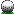HomeLeague Scoring ARRIS Golf League Scoring FormatThe new format is ARRIS Cup and is patterned after the FedEx Cup. Here is how it works- Points will be accumulated each round played over the course of the entire season- Finish position in a round will be based on net score.Regular league round, Points are awarded by finish position (everyone who plays scores points)1=100, 2=60, 3=38, 4=27, 5=22, 6=20, 7=18, 8=17, 9=16, 10=15, 11=14, 12=13, 13=12, 14=11, 15=11, 16=10, 17=10, 18=10, 19=9, 20=9, 21=9, 22=8, 23=8, 24=7, 25=7, 26=7, 27=7, 28=6, 29=6, 30=6, 31=5, 32=5, 33=5, 34=5, 35=5, 36=5, 37=5, 38=5, 39=5, 40=5 Ties will received the same points (e.g. 2 players tie for 3rd will both get 38 points).2 Majors during the season (one each half). Extra points are awarded for these rounds and consequently we will up the difficulty by playing back one tee. Points are awarded by finish position.1=200, 2=120, 3=76, 4=54, 5=44, 6=40, 7=36, 8=34, 9=32, 10=30, 11=28, 12=26, 13=24, 14=22, 15=21, 16=20, 17=19, 18=18, 19=17, 20=16, 21=16, 22=15, 23=15, 24=14, 25=14, 26=13, 27=13, 28=12, 29=11, 30=10The last 3 weeks of the season will be the Arris Cup Championship TournamentPlayoff Tournament 1. This Tournament will feature everyone in the league that has scored points. Points are awarded based on finish position. These points are added to the regular season total1=200, 2=120, 3=76, 4=54, 5=44, 6=40, 7=36, 8=34, 9=32, 10=30, 11=28, 12=26, 13=24, 14=22, 15=22, 16=20, 17=20, 18=20, 19=18, 20=18, 21=18, 22=16, 23=16, 24=14, 25=14, 26=14, 27=14, 28=12, 29=12, 30=12, 31=10, 32=10, 33=10, 34=10, 35=8, 36=8Playoff Tournament 2. This Tournament will feature the top 20 players (total points) after ArrisCup Playoff Tournament 1. Points are awarded based on finish position,1=200, 2=120, 3=76, 4=54, 5=44, 6=40, 7=36, 8=34, 9=32, 10=30, 11=28, 12=26, 13=24, 14=22, 15=22, 16=20, 17=20, 18=20, 19=18, 20=18, 21=18, 22=16, 23=16, 24=14, 25=14, 26=14, 27=14, 28=12, 29=12, 30=12, 31=10, 32=10, 33=10, 34=10, 35=8, 36=8Arris Cup Championship (final round). The top 10 players (total points) after ArrisCup Playoff Tournament 2 will vie for the title. Players are allocated seed points based on their position (based on total points) through Tournament 2. Players start the Championship with only their seed points1=2000, 2=1800, 3=1520, 4=1296, 5=1280, 6=1120, 7=960, 8=800, 9=640, 10=480, 11=384, 12=368, 13=350, 14=330, 15=310, 16=290 After the round points are awarded by finish position (net score)1=2000, 2=1200, 3=760, 4=540, 5=440, 6=400, 7=360, 8=340, 9=320, 10=300, 11=280, 12=260Championship round points are added to the player's seed points and the player with the most points is the Arris Lisle Golf League Champion.